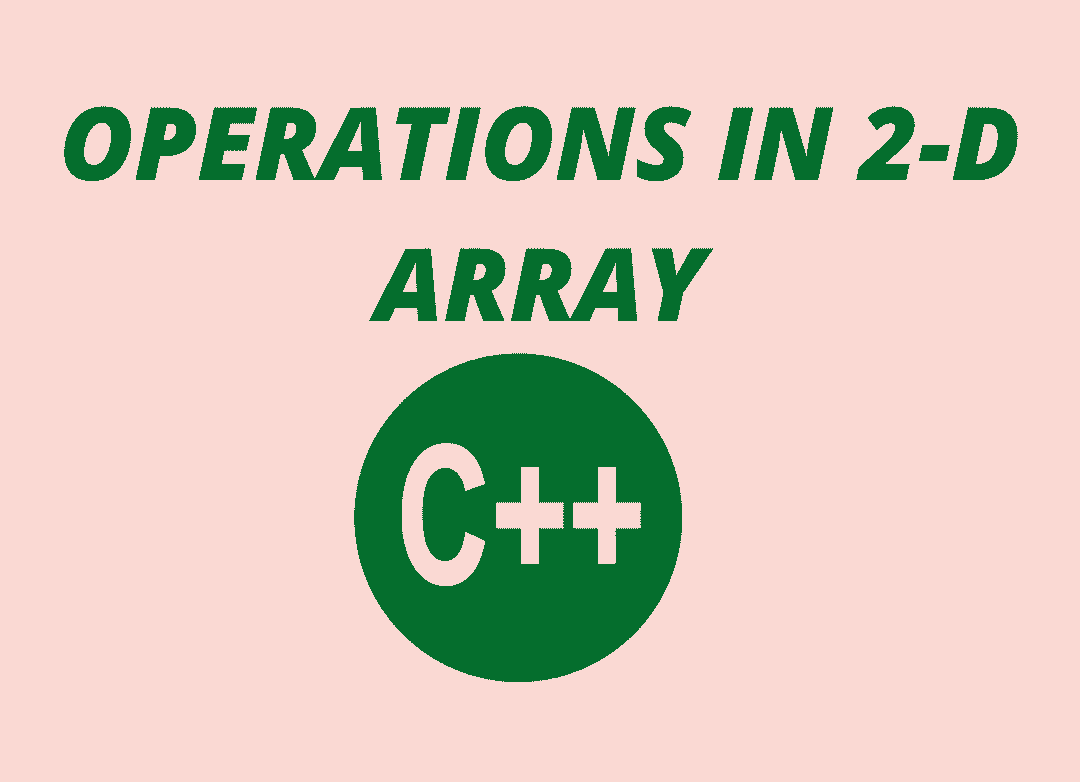December 6, 2023# SUM OF TWO ARRAYS (2-D arrays)

Hey guys, what is up. In this particular post, we will be discussing what is a two-dimensional array in c. Also, about how we can declare an array, initialize the value to an array. Also, about the sum of two arrays.

Updated on 1/10/2021

KEY POINTS

## TWO-DIMENSIONAL ARRAY:

DECLARATION Datatype arrayname [row][column];

Here, the data type is the data type of the element that will be stored in the array and the array name is the name of the array formed.

Example – int A ;

Here the subscript 2 shows the number of strings that we want our array to store in it and subscript 3 shows the length of each string. This is static memory allocation. We will be giving 2*3 memory locations to the array to store elements in it.

INITIALIZATION datatype arrayname [row][column] = {values};

Example – int A  = {{10,20,30}, {40,60,80}};

## PROGRAM TO INITIALIZE TWO-DIMENSIONAL ARRAY:

In initialization, firstly we will take input of the elements that we want to store in our array one by one. We will be using variable ‘i’ for rows and variable ‘j’ for columns for the working of loops. Then we will print the matrix drawn out of those elements in the order which is taken (like rows = 2 and columns = 3).

#include<stdio.h>

int main()

{

int arr;

int i, j;

for(i=0; i<2; i++)

{

for(j=0;j<3;j++)

{

printf(“Enter value for arr[%d][%d]:”, i, j);

scanf(“%d”, &arr[i][j]);

}

}

printf(“Two Dimensional array elements:\n”);

for(i=0; i<2; i++)

{

for(j=0;j<3;j++)

{

printf(“%d “, arr[i][j]);

if(j==2)

{

printf(“\n”);

}

}

}

return 0;

}

OUTPUT –

## SUM OF TWO ARRAYS:

In this question, we will be taking the sum of the two 2 dimensional arrays. First, we will be taking 3 arrays (array1[i][j], array2[i][j], array3[i][j]). In this, array3[i][j] Is the 3rd array that we will be taking as the sum of the other two arrays. Then we will be using variable ‘i’ for rows and ‘j’ for columns for working of loops. Then we will take input from the user for the elements of array 1 and then array 2 serial wises and then we will be showing the overall sum of the two arrays.

#include <stdio.h>

int main()

{

float array1, array2, array3;

int i, j;

printf(“Enter elements for array1 \n”);

for(i=0; i<2; ++i)

for(j=0; j<2; ++j)

{

printf(“Enter a %d%d: “, i+1, j+1);

scanf(“%f”, &array1[i][j]);

}

printf(“Enter elements for array2\n”);

for(i=0; i<2; ++i)

for(j=0; j<2; ++j)

{

printf(“Enter b %d%d: “, i+1, j+1);

scanf(“%f”, &array2[i][j]);

}

for(i=0; i<2; ++i)

for(j=0; j<2; ++j)

{

array3[i][j] = array1[i][j] + array2[i][j];

}

printf(“\n Overall Sum Of full Matrix is: \n”);

for(i=0; i<2; ++i)

for(j=0; j<2; ++j)

{

printf(“%.1f\t”, array3[i][j]);

if(j==1)

printf(“\n”);

}

return 0;

}

OUTPUT –

## DIFFERENCE OF TWO ARRAYS:

The operation of the difference of two arrays is exactly the same as the previous operation of the sum of two arrays just we have to change the sign of adding to subtracting and the overall working of this program will be going the same.

#include <stdio.h>

int main()

{

float array1, array2, array3;

int i, j;

printf(“Enter elements for array1 \n”);

for(i=0; i<2; ++i)

for(j=0; j<2; ++j)

{

printf(“Enter a %d%d: “, i+1, j+1);

scanf(“%f”, &array1[i][j]);

}

printf(“Enter elements for array2\n”);

for(i=0; i<2; ++i)

for(j=0; j<2; ++j)

{

printf(“Enter b %d%d: “, i+1, j+1);

scanf(“%f”, &array2[i][j]);

}

for(i=0; i<2; ++i)

for(j=0; j<2; ++j)

{

array3[i][j] = array1[i][j] – array2[i][j];

}

printf(“\n Overall Difference Of full Matrix is: \n”);

for(i=0; i<2; ++i)

for(j=0; j<2; ++j)

{

printf(“%.1f\t”, array3[i][j]);

if(j==1)

printf(“\n”);

}

return 0;

}

OUTPUT –

## SUM OF DIAGONAL ELEMENTS:

For finding the sum of diagonal elements of the array, first, we will be taking input from the user the number of rows and columns of the matrix. Then we will be taking the input of elements that the user wants to store in an array and then we will print the array in matrix form and then it will be showing the sum of the diagonal elements of that matrix.

#include<stdio.h>

void main()

{

int mat;

int i,j,row,col,sum=0;

printf(“Enter the number of rows and columns for 1st matrix\n”);

scanf(“%d%d”,&row,&col);

printf(“Enter the elements of the matrix\n”);

for(i=0;i<row;i++)

{

for(j=0;j<col;j++)

{

scanf(“%d”,&mat[i][j]);

}

}

printf(“The matrix\n”);

for(i=0;i<row;i++)

{

for(j=0;j<col;j++)

{

printf(“%d\t”,mat[i][j]);

}

printf(“\n”);

}

for(i=0;i<row;i++)

{

for(j=0;j<col;j++)

{

if(i==j)

{

sum=sum+mat[i][j];

}

}

}

printf(“The sum of diagonal elements of a square matrix = %d\n”,sum);

}

OUTPUT –

In the upcoming post, you will be getting information related to some more operations that are performed in a two-dimensional array and also about how to transpose an array. If you haven’t read about What is a two-dimensional array then go and read this. Also, stay tuned for the upcoming posts. You can also refer geeks for geeks.#### Sneha Shukla

View all posts by Sneha Shukla →

## 3 thoughts on “SUM OF TWO ARRAYS (2-D arrays)”

1.chitra munjal says:

beautifully designed website also very helpful and information !!!☺️☺️

1.Prakhar Jain says:

Very crisp and on point, exactly what I needed. 👍

2.Naman Kumar says:

Op blog! helped me get a clearer picture